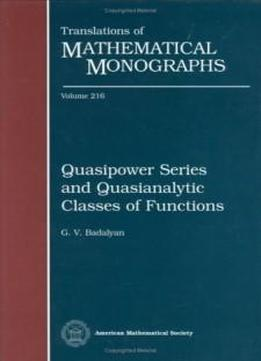# Quasipower Series And Quasianalytic Classes Of Functions (translations Of Mathematical Monographs) by G. V. Badalyan / 2002 / English / PDF, DjVu

In this book, G. V. Badalyan addresses the fundamental problems of the theory of infinitely-differentiable functions using the theory of functions of quasianalytic classes. A certain class of functions \$C\$ on an interval is called quasianalytic if any function in \$C\$ is uniquely determined by the values of its derivatives at any point. The obvious question, then, is how to reconstruct such a function from the sequence of values of its derivatives at a certain point. In order to answer that question, Badalyan combines a study of expanding functions in generalized factorial series with a study of quasipower series. The theory of quasipower series and its application to the reconstruction problem are explained in detail in this research monograph. Along the way other, related problems are solved, such as Borel's hypothesis that no quasianalytic function can have all positive derivatives at a point. Originally published in Russian, this English translation contains additional material that treats the problems of classification of infinitely-differentiable functions, conditions for absolute convergence of quasipower series in terms of the functions that generate them, and the possibility of representing analytic functions by quasipower series in non-circular domains. While the treatment is technical, the theory is developed chapter by chapter in detail, and the first chapter is of an introductory nature. The quasipower series technique explained here provides the means to extend the previously known results and elucidates their nature in the most relevant manner. This method also allows for thorough investigation of numerous problems of the theory of functions of quasianalytic classes by graduate students and research mathematicians.

In this book, G. V. Badalyan addresses the fundamental problems of the theory of infinitely-differentiable functions using the theory of functions of quasianalytic classes. A certain class of functions \$C\$ on an interval is called quasianalytic if any function in \$C\$ is uniquely determined by the values of its derivatives at any point. The obvious question, then, is how to reconstruct such a function from the sequence of values of its derivatives at a certain point. In order to answer that question, Badalyan combines a study of expanding functions in generalized factorial series with a study of quasipower series. The theory of quasipower series and its application to the reconstruction problem are explained in detail in this research monograph. Along the way other, related problems are solved, such as Borel's hypothesis that no quasianalytic function can have all positive derivatives at a point. Originally published in Russian, this English translation contains additional material that treats the problems of classification of infinitely-differentiable functions, conditions for absolute convergence of quasipower series in terms of the functions that generate them, and the possibility of representing analytic functions by quasipower series in non-circular domains. While the treatment is technical, the theory is developed chapter by chapter in detail, and the first chapter is of an introductory nature. The quasipower series technique explained here provides the means to extend the previously known results and elucidates their nature in the most relevant manner. This method also allows for thorough investigation of numerous problems of the theory of functions of quasianalytic classes by graduate students and research mathematicians.

views: 355